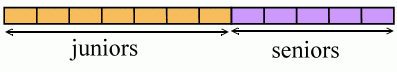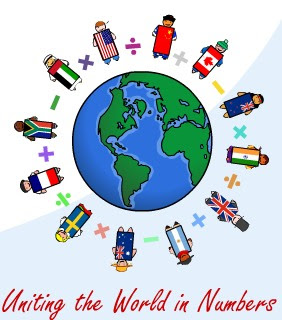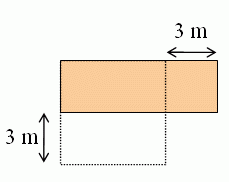## Posts

Showing posts from February, 2008

### Foerster's Algebra 1 plus a Home Study Companion

Here's another alternative for an algebra 1 course for homeschooled students: Algebra 1 by Paul Foerster (the textbook), and accompanying Home Study Companion video lessons made by David Chandler. I've just written an in-depth review of both .

### A bar diagram to solve a ratio problemDave at MathNotations had an interesting ratio problem : In Virtual HS, the ratio of the number of juniors to seniors is 7:5. The ratio of (the number of) junior males to junior females is 3:2. The ratio of senior males to senior females is 4:3. What is the ratio of junior males to senior females? He asked if it can be solved using "Singapore" style bar model. I'm not sure if this is exactly how they'd do it, but this is how I'd do it... so here goes. After I made the diagrams, I soon saw that Dave's numbers are two awkward; the bar diagram drawing would get too messy because we'd need to divide it into too tiny parts to see anything. BUT... you probably know about the PROBLEM SOLVING STRATEGY called "solve an easier problem". My agenda is therefore: show how to solve a few related simple ratio problems using the bar diagram solve a variant of the original problem (with friendly numbers) solve the original problem. 1. Here's a ba

### Notice

For those of you who're waiting... LightBlue 4th grade is just days from being finished. Right now I'm putting finishing touches on the lists of web resources I've added to each chapter. Just about everything else is ready.

### Concept of average

I felt like giving away something again to help all of you who need math lessons... This one is taken from Math Mammoth Division 2 book and is a lesson on the concept of average... meant for initial teaching for 4th-5th graders who have mastered long division. I've written it quite recently, and in fact improved it just last night by adding the bar graph problems into it. (That seems to be the "way of life" with these books: I constantly keep tweaking and improving them as I learn. Which means constant uploading of files here and there... keeping our bandwidth usage large...) The lesson mainly uses word problems, BTW. Please post your comments below; I'm anxious to hear them! Right click and choose save: Average_from_Math_Mammoth_Division_2.pdf

### Carnival of Homeschooling, Schoolhouse Edition

There's lots to read and enjoy at the newest carnival of homeschooling . I enjoyed the old schoolhouse photos as well! I know my dad attended a fairly small village school; maybe it was similar to these schoolhouses. One math-related pick from the carnival: Griddlers make great “paint by number” puzzles for kids of all ages - Sol from Wild About Math shares some great number puzzles where you color in a picture based on number clues.

### Learn to recognize numbers game for preschoolers

I just wanted to share a little "game" that helped my 3-year old preschooler to recognize her numbers. It's SO simple! Learn to recognize numbers - simple game Basically you just pick numbers, calling out their names, and taking turns.

### Pi is a ratio, yet irrational?

Here's an interesting dilemma: We know Pi is an irrational number; mathematicians have proven it to be so. But its definition says that it is a RATIO of the circumference and the diameter of any circle. Now, when you divide a rational number by another rational number, you get a rational number. Doesn't this seem like a contradiction? In the words of a certain visitor to my site: In the equation where the circumference is divided by the diameter, when the circumference and diameter are rational values, why is it that the quotient can be an irrational quantity? The solution First of all, like a commenter pointed out, Pi being a ratio of two numbers does not mean it is rational. Pi has been established as irrational, and we know Pi = C/d, where C is the circumference and d is the diameter of some circle. It follows that either C or d or both have to be irrational! This is kind of amazing to think about, but it's true: for any circle, either the circumference, or

### The World Math Day ChallengeI got word about this nice math event. In World Math Day Challenge (held on March 5), students from across the globe will be uniting online to play each other in real time mental arithmetic games. These are tailored to the students' levels so students of various ages can participate. They are expecting more than 1 million students from over 100 countries! It is one of the world's largest participation events. World Math Day is free of charge for both schools and students. All you need is Internet access. And, there are even prizes! More information may be found at www.WorldMathDay.com . This is a nice opportunity to do something fun with math, and at the same time connect with other kids, even across the globe, without leaving your home. You can register already; the event is on March 5.

### Shorter-wider table problemI failed to publish this the other day since Blogger was acting up. I'll try it now. A rectangular kitchen table is three times as long as it is wide. If it were 3 m shorter and 3 m wider it would be a square. What are the dimensions of the rectangular table? 1) You can solve this using algebra: set width to be x, the length is then 3x. We know these two will be equal if the former is increased by 3, and the latter is decreased by 3: x + 3 = 3x - 3 2x = 6 x = 3. The dimensions are 3 and 9. But since this is supposed to be a 6th grade problem, surely we can find another way to solve it, as well. 2) Think of the two quantities length and width as just numbers. If you reduce one by 3, and increase the other by 3, they will "meet" or be the same. Below, I've drawn the two numbers as lines; you could use bars. length |---------------------------------| width |----------| If I decrease the length by 3 and increase the width by 3, they'll be equal: length |----------

### Longer-wider problem to solve

I took another problem from the same collection as before , this time for 6th grade. It reads: A rectangular kitchen table is three times as long as it is wide. If it were 3 m shorter and 3 m wider it would be a square. What are the dimensions of the rectangular table? I'll let you try it first, but don't post the solution as a comment into this post. Let others try solve it too. (You can get an extra point for answering this: Is this a realistic kitchen table?)

### Blogged math blogs

I got word that folks at Blogged.com had rated a bunch of math blogs, and placed mine in the top ten, with rating 8.6 (out of 10). They evaluate blogs based on the following criteria: Frequency of Updates, Relevance of Content, Site Design, and Writing Style. So here is a list of mathematics blogs , rated in a scale 0-10. The list does resemble the blogrolls that I've seen on other math blogs... If you're a math blogger, go find yours!